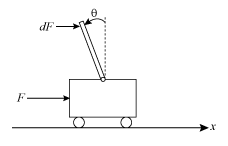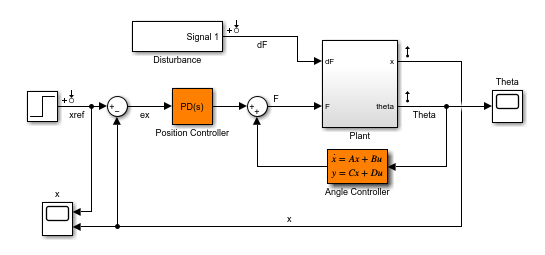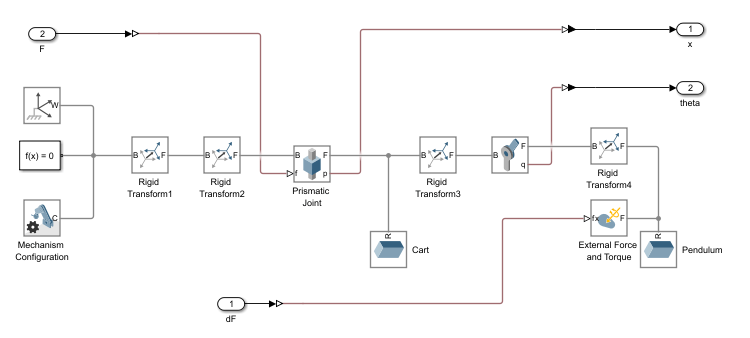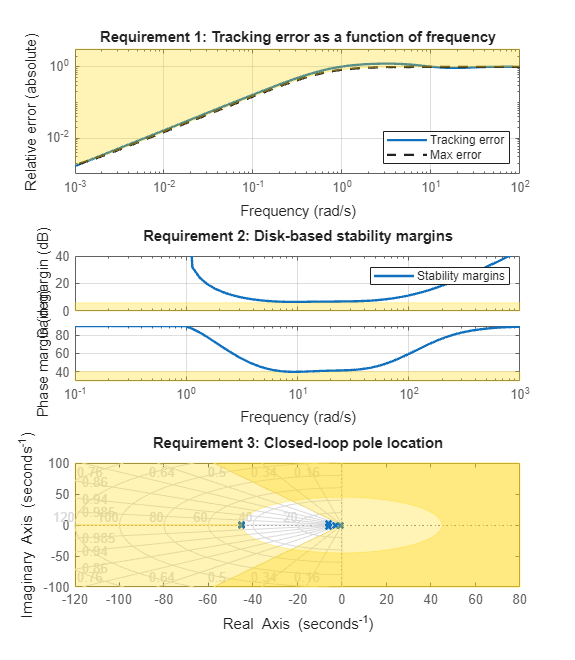# Control of an Inverted Pendulum on a Cart

This example uses systune to control an inverted pendulum on a cart.

### Pendulum/Cart Assembly

The cart/pendulum assembly is depicted in Figure 1 and modeled in Simulink using Simscape Multibody.Figure 1: Inverted pendulum on a cartFigure 2: Simscape Multibody model

This system is controlled by exerting a variable forceon the cart. We need to keep the pendulum upright while moving the cart to a new position or when the pendulum is nudged forward (impulse disturbance).

### Control Structure

The upright position is an unstable equilibrium for the inverted pendulum. The unstable nature of the plant makes the control task more challenging. For this example, we use the following two-loop control structure:

open_system('rct_pendulum.slx') set_param('rct_pendulum','SimMechanicsOpenEditorOnUpdate','off');The inner loop uses a second-order state-space controller to stabilize the pendulum in its upright position (control), while the outer loop uses a Proportional-Derivative (PD) controller to control the cart position. Note that we use a PD rather than PID controller because the plant already provides some integral action.

### Design Requirements

Use TuningGoal requirements to specify the desired closed-loop behavior. Specify a response time of 3 seconds for tracking a setpoint change in cart position.

% Tracking of x command req1 = TuningGoal.Tracking('xref','x',3); 

To adequately reject impulse disturbanceson the tip of the pendulum, use an LQR penalty of the formthat emphasizes a small angular deviationand limits the control effort.

% Rejection of impulse disturbance dF Qxu = diag([16 1 0.01]); req2 = TuningGoal.LQG('dF',{'Theta','x','F'},1,Qxu); 

For robustness, require at least 6 dB of gain margin and 40 degrees of phase margin at the plant input.

% Stability margins req3 = TuningGoal.Margins('F',6,40); 

Finally, constrain the damping and natural frequency of the closed-loop poles to prevent jerky or underdamped transients.

% Pole locations MinDamping = 0.5; MaxFrequency = 45; req4 = TuningGoal.Poles(0,MinDamping,MaxFrequency); 

### Control System Tuning

The closed-loop system is unstable for the initial values of the PD and state-space controllers (1 and, respectively). You can use systune to jointly tune these two controllers. Use the slTuner interface to specify the tunable blocks and register the plant input F as an analysis point for measuring stability margins.

ST0 = slTuner('rct_pendulum',{'Position Controller','Angle Controller'}); addPoint(ST0,'F'); 

Next, use systune to tune the PD and state-space controllers subject to the performance requirements specified above. Optimize the tracking and disturbance rejection performance (soft requirements) subject to the stability margins and pole location constraints (hard requirements).

rng(0) Options = systuneOptions('RandomStart',5); [ST, fSoft] = systune(ST0,[req1,req2],[req3,req4],Options); 
Final: Soft = 1.38, Hard = 0.99851, Iterations = 313 Final: Soft = 1.44, Hard = 0.99925, Iterations = 157 Final: Soft = 1.44, Hard = 0.99858, Iterations = 300 Final: Soft = 1.27, Hard = 0.99402, Iterations = 265 Final: Soft = 1.44, Hard = 0.99986, Iterations = 277 Final: Soft = 1.4, Hard = 0.99855, Iterations = 257 Some closed-loop poles are marginally stable (decay rate near 1e-07) 

The best design achieves a value close to 1 for the soft requirements while satisfying the hard requirements (Hard<1). This means that the tuned control system nearly achieves the target performance for tracking and disturbance rejection while satisfying the stability margins and pole location constraints.

### Validation

Use viewGoal to further analyze how the best design fares against each requirement.

figure('Position',[100 100 575 660]); viewGoal([req1,req3,req4],ST)These plots confirm that the first two requirements are nearly satisfied while the last two are strictly enforced. Next, plot the responses to a step change in position and to a force impulse on the cart.

T = getIOTransfer(ST,{'xref','dF'},{'x','Theta'}); figure('Position',[100 100 650 420]); subplot(121), step(T(:,1),10) title('Tracking of set point change in position') subplot(122), impulse(T(:,2),10) title('Rejection of impulse disturbance')The responses are smooth with the desired settling times. Inspect the tuned values of the controllers.

C1 = getBlockValue(ST,'Position Controller') 
C1 = s Kp + Kd * -------- Tf*s+1 with Kp = 5.96, Kd = 2.06, Tf = 0.0534 Name: Position_Controller Continuous-time PDF controller in parallel form. 
C2 = zpk(getBlockValue(ST,'Angle Controller')) 
C2 = -1596.7 (s+12.9) (s+4.396) -------------------------- (s+134.3) (s-14.18) Name: Angle_Controller Continuous-time zero/pole/gain model. 

Note that the angle controller has an unstable pole that pairs up with the plant unstable pole to stabilize the inverted pendulum. To see this, get the open-loop transfer at the plant input and plot the root locus.

L = getLoopTransfer(ST,'F',-1); figure; rlocus(L) set(gca,'XLim',[-25 20],'YLim',[-20 20])To complete the validation, upload the tuned values to Simulink and simulate the nonlinear response of the cart/pendulum assembly. A video of the resulting simulation appears below.

writeBlockValue(ST)Figure 3: Cart/pendulum simulation with tuned controllers.

Close the model after simulation.

set_param('rct_pendulum','SimMechanicsOpenEditorOnUpdate','on'); close_system('rct_pendulum',0);